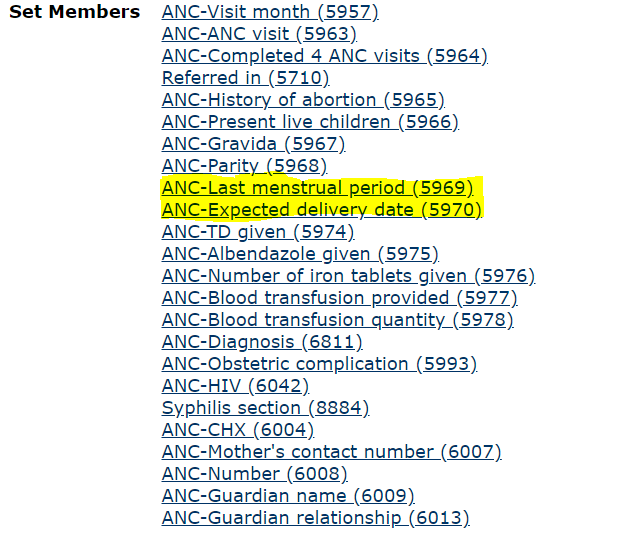# Bahmni - EDD Calculate

I try to auto calculate EDD but does not work. Some Screenshot of work are attached here.

# concepts are given below#code are given below on file : Karnali_Implementation\openmrs\obscalculator\BahmniObsValueCalculator.groovy

``````    BahmniObservation lmpObservation = find("ANC-Last menstrual period", observations, null)
def calculatedConceptName = "ANC-Expected delivery date"
if (hasValue(lmpObservation)) {
parent = obsParent(lmpObservation, null)
def calculatedObs = find(calculatedConceptName, observations, null)

Date obsDatetime = getDate(lmpObservation)

LocalDate edd = new LocalDate(lmpObservation.getValue()).plusMonths(9).plusWeeks(1)
if (calculatedObs == null)
calculatedObs = createObs(calculatedConceptName, parent, bahmniEncounterTransaction, obsDatetime) as BahmniObservation
calculatedObs.setValue(edd)
return
} else {
def calculatedObs = find(calculatedConceptName, observations, null)
if (hasValue(calculatedObs)) {
voidObs(calculatedObs)
}
}
``````

# result (dont _work)

Hmm,

1. have you checked the default groovy scripts for BMI Status calc?
2. Are you sure your EDD is properly calculated and saved onto DB? Try putting some log statements
3. Lastly, why not just use Form2, you can do all these using javascript - will give you faster feedback.